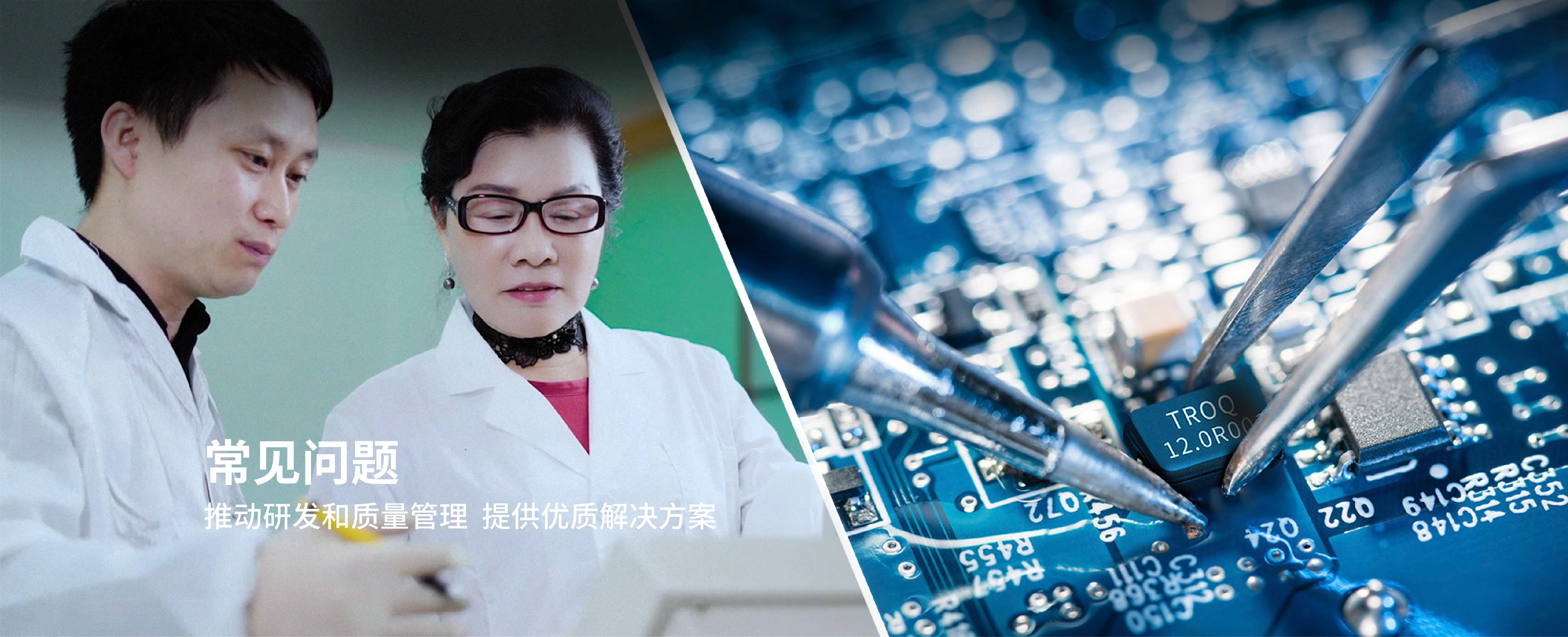• All

• Product Problems

• Technical Problems

• Q

Why use crystal shakers as oscillators?

Because the oscillation frequency generated by it is more accurate and stable than other oscillation components, and it is easy to design and simple circuit is its biggest advantage

• Q

Why crystal vibrator and quartz oscillator can not fall to?

Because the wafer thickness of crystal vibrator is very thin. Take 25Mhz as an example, its thickness is about 0.0668mm, if it is dropped it will cause the wafer to break and some other factors

• Q

What effect does a low power supply design have on an oscillator?

Simply put, when designing a high-frequency OSCILLATOR, it will face low high-frequency gain and high CRYSTAL equivalent resistance of the amplifier.

• Q

What is PPM and how is it calculated?

Part Per Million means the frequency hopping value in parts per million; the actual frequency minus the destination frequency and divided by the destination frequency and moving the decimal point back six digits is the ppm value. Example: The destination frequency is 25.000000MHz, the actual frequency is 25.000015MHz (25.000015-25.000000)/25.000000=0.0000006 Move the decimal point back six digits to 0.6ppm.

• Q

What is the meaning of CL in the manufacturer's specification?

It refers to the load capacitance added when making the oscillator, so when used in the oscillation circuit should add equal capacitance, so that the oscillation frequency will be accurate.

• Q

What is the meaning of CL in the manufacturer's specification?

It refers to the load capacitance added when making the oscillator, so when used in the oscillation circuit should add equal capacitance, so that the oscillation frequency will be accurate.

• Q

Why use crystal shakers as oscillators?

Because the oscillation frequency generated by it is more accurate and stable than other oscillation components, and it is easy to design and simple circuit is its biggest advantage

• Q

Why crystal vibrator and quartz oscillator can not fall to?

Because the wafer thickness of crystal vibrator is very thin. Take 25Mhz as an example, its thickness is about 0.0668mm, if it is dropped it will cause the wafer to break and some other factors

• Q

What effect does a low power supply design have on an oscillator?

Simply put, when designing a high-frequency OSCILLATOR, it will face low high-frequency gain and high CRYSTAL equivalent resistance of the amplifier.

• Q

What is PPM and how is it calculated?

Part Per Million means the frequency hopping value in parts per million; the actual frequency minus the destination frequency and divided by the destination frequency and moving the decimal point back six digits is the ppm value. Example: The destination frequency is 25.000000MHz, the actual frequency is 25.000015MHz (25.000015-25.000000)/25.000000=0.0000006 Move the decimal point back six digits to 0.6ppm.